Design of Multichannel Sample Rate Convertor | OMICS International
Journal of Electrical & Electronic Systems

# Design of Multichannel Sample Rate Convertor

Jain V* and Agrawal N

Department of Electronics and Communication, College of Technology and Engineering, MPUAT, Udaipur, India

*Corresponding Author:
Jain V
Department of Electronics and Communication
College of Technology and Engineering MPUAT
Udaipur, India
Tel: 0294-247-1302
E-mail: [email protected]

Received Date: November 12, 2015; Accepted Date: December 29, 2015; Published Date: January 01, 2016

Citation: Jain V, Agrawal N (2016) Design of Multichannel Sample Rate Convertor. J Electr Electron Syst 5:168. doi:10.4172/2332-0796.1000168

Copyright: © 2016 Jain V, et al. This is an open-access article distributed under the terms of the Creative Commons Attribution License, which permits unrestricted use, distribution, and reproduction in any medium, provided the original author and source are credited.

Visit for more related articles at Journal of Electrical & Electronic Systems

#### Abstract

The multiobjective design of multichannel sample rate convertor using Genetic optimization technique is considered in this paper. This new optimization tool is based on mechanism of biological evolution. It is characterized by design of natural system retaining its robustness and adaption properties of natural systems. The objectives of multichannel sample rate convertor design include matching some desired response while having minimum linear phase; hence, reducing the time response, constant group delay, increasing bandwidth. Genetic optimization technique is also used for reducing the power consumption of multichannel sample rate convertor by optimization of coefficient of filter by scaling which are used in implementation of multichannel sample rate convertor design in FPGA implementation. After applying genetic algorithm 1 to 128 channel sample rate convertor bandwidth increased by 150%, power reduced by 62% to 85%, dynamic power reduced by 31% to 54% of conventional sample rate convertor, constant and less group delay, linear phase response, reducing time response. In an extended work the authors have tried and successfully executed the model and system upto 128 channels. The proposed model is first designed on simulink platform using Xilinx Blockset and then it is transferred on FPGA platform using system generator. The complete circuit is synthesized, implemented, simulated using Xilinx design suite.

#### Keywords

Multiobjective design; Genetic optimization technique; Magnitude response; Minimum linear phase; Group delay; Pipelines

#### Introduction

The full utilization and practical realization of Multichannel sample rate convertor is restricted by increased power consumption and resource utilization of the interpolator and decimator design in the context of contemporary wireless broad band standards using. So we are trying to make all the multimedia devices compatible with all the wireless communication multiple standards. For that purpose we are about to develop sample rate converter which will provide the flexibility to change its sampling rate according to requirement of the different wireless multiple standards. The second challenge of resampler design is that the numbers of channels are limited. In such a case, we have to develop multichannel interpolator and decimator of high performance low power consumption with near optimal performance.

Generally conventional interpolator and decimator implemented by using direct form FIR filter structure. The problem with Implementing Sample Rate Convertor using direct form architecture was that filter length linearly increases with the decimation and Interpolation rate. Therefore resource Utilization also increases; this in turn increases Power consumption, Area requirement and Delay of the sample rate convertor. To overcome these problem CIC FIR filter structure to implement sample rate convertor. CIC filters can efficiently perform either decimation or interpolation, with two complementary structures being employed to implement these functions. The problem with implementation of Sample Rate Convertor using CIC filter was that CIC filter was used only for narrowband signals. So it would not be used for implementation of sample rate convertor for large bandwidth. MAC architecture was an efficient solution developed as a sample rate convertor for large bandwidth signal.

MAC Architecture can also be used for increase bandwidth of the input signal. This application note deals with single MAC filters, as the low sample rates at which the FIR filters operate allow high clock-persample ratios, allowing many taps to be calculated in a single multiplier in each sample period. Limitation of MAC Architecture is not support for large change in sampling rate conversion but allow large bandwidth signal. Our purpose model CMFIR filter is an efficient solution of that problem. On CMFIR implement cascading of CIC and MAC FIR filter [1,2].

We apply genetic algorithm on coefficient of CMFIR filter to achieve desired frequency and magnitude response while having minimum linear phase; hence, reducing the time response, constant group delay, increasing bandwidth. Genetic optimization technique is also used for reducing the power consumption of multichannel sample rate convertor by optimization of coefficient of filter by scaling which are used in implementation of multichannel sample rate convertor design in FPGA implementation.

#### Problem Formulations

1. Number of Input Channels limited the total filter length grows linearly with the decimation rate. So that resource Utilization also increases.

2. To achieve change in high sampling rate is not possible with existing polyphase structure. It is not reliable.

3. Sample rate Convertor is implemented by using the Cascaded Integrator Comb (CIC) filter for narrowband.

4. Processing speed is very low.

5. Power consumption is very large.

6. Current commercial Sample Rate Convertor typically supports 32 channels.

7. We cannot change the sampling during the run time.

8. Non Linear Phase response of sample rate convertor.

9. Group Delay of sample rate convertor is very high.

#### Objective

1. To design and optimize CIC FIR filter to prevent aliasing.

2. To design and optimize MAC FIR filter to smoothen out the signal transitions.

3. Cascading of CIC and MAC FIR filter to design and optimize CM FIR filter.

4. To design and optimize suitable decimator and interpolator using CM FIR filter, delay and advanced units.

5. Assembling of single channel sample rate convertor using decimator and interpolator designed in objective (d).

6. To develop and optimize 8 channel sample rate convertor using multiplexer unit and single channel sample rate convertor.

7. To develop and optimize 16, 32, 64, 128 channel sample rate convertor using 8 channel sample rate convertor and multiplexer.

8. FPGA implementation of multichannel sample rate convertor and performance analysis.

#### Genetic Algorithms

Genetic Algorithm is very flexible, no problem specific, and robust. It can explore multiple regions of the parameter space for solutions simultaneously. Owing to the heuristic nature of GAs, arbitrary constraints can be imposed on the objective function without increasing the mathematical complexity of the problem. Multiple objective functions can be optimized simultaneously.

Gene: A single encoding of part of the solution space, i.e., either single bits or short blocks of adjacent bits that encode an element of the candidate solution.

Chromosome: A string of genes that represents a solution.

Population: The number of chromosomes available to test. Start with a population of candidate solutions

Variation: Introduce variation by applying two operators: crossover and mutation

Survival of the fittest: Use a fitness criterion to bias the evolution towards desired features.

Representation:

1. GAs on primarily two types of representations:

2. Binary Coded [0110, 0011, 1101,]

3. Real Coded [13.2, -18.11, 5.72,]

4. Binary-Coded (genotype) GAs must decode a chromosome into a real value (phenotype), for evaluating the fitness value.

5. Real-Coded GAs can be regarded as GAs that operates on the actual real value (phenotype).

6. For Real-Coded GAs, no genotype-to-phenotype mapping is needed.

Selection: A proportion of the existing population is selected to bread a new bread of generation.

Crossover: It is a genetic operator that combines (mates) two individuals (parents) to produce two new individuals (Childs). The idea behind crossover is that the new chromosome may be better than both of the parents if it takes the best characteristics from each of the parents.

Mutation: It is a genetic operator used to maintain genetic diversity from one generation of a population of chromosomes to the next.

Steps of genetic algorithm

The genetic algorithm loops over an iteration process to make the population evolves (Figure 1). Each consists of the Following steps:

1. Selection: The first step consists in selecting individuals for reproduction. This selection is done

Randomly with a probability depending on the relative fitness of the individuals so that best ones are often Chosen for reproduction than poor ones.

2. Reproduction: In the second step, offspring are bred by the selected individuals. For generating new chromosomes, the algorithm can use both recombination and mutation.

3. Evaluation: Then the fitness of the new chromosomes is evaluated.

4. Replacement: During the last step, individuals from the old population are killed and replaced by the new ones .

#### Methodology to Develop Multichannel Sample Rate Convertor Approach

A multichannel sample rate convertor based solution is proposed to make all the multimedia devices compatible with all the wireless communication multiple standards. The Multichannel sample rate convertor time response must be included in the requirements. On one hand, the time domain requirement where both a high speed and accurate system response are needed. On the other hand, the frequency domain requirements (DC, sub-synchronous and harmonic components elimination) which are the magnitude response within small bandwidth including sharp frequency edges as well as an approximately constant group delay in this band are required too. Usually the best optimum value of all the objective functions of this multichannel sample rate convertor design can be obtained for some values of design variables. A compromise or a trade-off between the objective functions must be made to achieve a satisfactory multichannel sample design [1,2].

The considered CMFIR multichannel sample rate convertor satisfies four multi-objective functions. These functions are: 1) Meet a specified or a desired response specification; 2) An approximately constant group delay; and 3) A minimum time response or settling time which involves a minimum phase or a group delay. 4) For reducing the power consumption.

Transfer function of multichannel sample rate convertor

The two basic building blocks of a CIC filter are an integrator and a comb. CIC filters can efficiently perform either decimation or interpolation, with two complementary structures being employed to implement these functions. Decimation requires a cascade of a number of integrator units, followed by a down-sampling stage and finally a cascade of the comb filter units. Conversely, interpolation cascades several comb filters with an up-sampler and several integrators. A comb filter running at the high sampling rate, fs, for a rate change of RM is an odd symmetric FIR filter described by .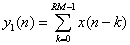(1)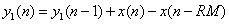(2)

The second equality corresponds to a comb is given by: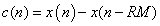(3)

Comb followed by an integrator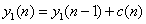(4)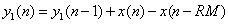(5)

Taking Z transform of the equation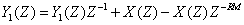(6)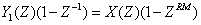(7)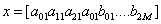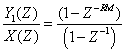(8)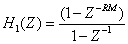(9)

To generate parameterizable, high-performance and area-efficient filter modules utilizing the Multiply-Accumulate (MAC) architecture. Multiple MACs can be used in achieving higher performance filter requirements, such as longer filter coefficients, higher throughput, or increased channel support. Output of the MAC FIR filter is given by: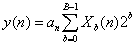(10)

Taking Z Transform: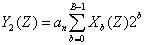(11)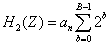(12)

Where

y(n) = Output of the MAC filters

an = Coefficient of the filter

xb = The bth bit of x[n].

B = The Total input width.

Cascaded multiple architecture finite impulse response (CMFIR) filter will be implemented by cascading of CIC filter and MAC architecture. The CMFIR filter (CMFIR) is an interpolating and decimator low-pass FIR filter. It provides a further increase in sample rate, reducing the resources requirements on the CIC and limiting the number of stages required, while also providing moderate pass band filtering of the QPSK modulated signal (although this requirement is less stringent where an effective QPSK modulator with good pulseshaping properties has been used).

Final Response of CMFIR Filter: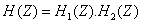(13)

Magnitude response objective function

The amplitude and the phase responses of multichannel

Sample rate convertor are given by: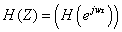(14)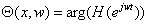(15)

Where w is the frequency and x is a column vector with 2M + 2N + 1 components, that is in Cartesian form .(16)

Group-delay and phase response objective function

The group delay is derived from the phase relation, as given in equation (11), and is defined as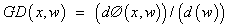(17)

Where is Ø(x,w) the phase response of the filter .

#### Genetic Algorithm Implementation Methodology

The design flow is highlighted in below (Figure 2). Here are the steps that we follow:

Optimization of power dissipation

1. Given set of design specification, program them into MATLAB.

2. Create an ideal response, group delay of the desired multichannel sample rate convertor.

3. Use the order estimation function provided by MALTAB for each type of multichannel sample rate convertor.

4. Design the GA multichannel sample rate filter using MATLAB multichannel sample rate convertor function.

5. Compare the response, phase response, group delay of our design with the ideal multichannel sample rate convertor .

Optimization of power dissipation

The coefficient optimization is done in two phases:

In the first phase, all the coefficients are scaled uniformly. The advantage of such an approach is that it does not affect the sample rate convertor characteristics in terms of pass band ripples and stop band attenuation and phase response. The sealing results in the same gain/ attenuation ratio.

In the second phase of optimization one coefficient is perturbed in the each iteration. In case of requirement to retain the linear phase characteristics, the coefficients are perturbed in pairs (Ai and An-1-i) so as to preserve coefficients symmetry. The selection of coefficient for perturbation and the amount of perturbation has the direct impact on overall optimization quality. Various strategies can be adopted for coefficient perturbation. The strategies adopted here include ‘Genetic Algorithms’. The Genetic Algorithms are the evolutionary algorithm which generates the random numbers and selects the best fit value according to the fitness function and search the whole space to find the global value [5-7].

#### Implementation

a) Develop the MATLAB Subscript.

b) Developed the model on simulink using Xilinx Blockset.

c) Generate the HDL code for that simulink model using Xilinx System Generator.

d) Implement this model on FPGA with help hardware description language using ISE Design Suite.

e) Estimation of power consumption using Xilinx PLAN AHEAD.

f) Estimation of Resources utilization on FPGA using Xilinx ISE Design Suite.

#### Simulation Result

Tables 1-8 indicates simulation results.

Number of Channel MAC(mw) CIC(mw) CMFIR without genetic algorithm(mw) CMFIR with genetic algorithm(mw)
1 429 63 62 61
2 536 70 69 68
4 762 91 89 87
8 802 129 127 118
16 881 204 196 171
32 1040 357 326 270
64 1222 489 465 403
128 1424 630 583 540

Table 1: Total Power consume in fractional sample rate convertor based on different architecture of FIR filter.

Number of Channel MAC(mw) CIC(mw) CMFIR without genetic algorithm (mw) CMFIR with genetic algorithm (mw)
1 33 17 16 15
2 42 24 23 22
4 56 45 43 41
8 95 82 80 71
16 173 156 148 123
32 329 306 276 221
64 508 436 413 353
128 706 574 529 489

Table 2: Dynamic Power consume in fractional sample rate convertor based on different architecture of FIR filter.

Number of Channel MAC(mw) CIC(mw) CMFIR without genetic algorithm (mw) CMFIR with genetic algorithm (mw)
1 396 46 46 46
2 494 46 46 46
4 706 46 46 46
8 707 47 47 47
16 708 48 48 48
32 711 51 50 50
64 714 53 52 50
128 718 56 54 51

Table 3: Static Power consume in fractional sample rate convertor based on different architecture of FIR filter.

Filter Structure Bandwidth(MHz)
CMFIR with Genetic Algorithm 309.6
CMFIR withoutGenetic Algorithm 260.82
MAC 202.66
CIC 123.54

Table 4: Bandwidth for data transfer of fractional sample rate convertor based on different architecture of FIR filter.

Architecture(Number of Channel) Number of Register
CIC (1) 2037
MAC(1) 1270
CMFIR Without Genetic Algorithm (1) 476
CMFIR With Genetic Algorithm (1) 472
CIC (2) 3991
MAC(2) 2476
CMFIR Without Genetic Algorithm (2) 934
CMFIR With Genetic Algorithm (2) 926
CIC (4) 7889
MAC(4) 4888
CMFIR Without Genetic Algorithm (4) 1856
CMFIR With Genetic Algorithm (4) 1850
CIC (8) 15715
MAC(8) 9712
CMFIR Without Genetic Algorithm (8) 3682
CMFIR With Genetic Algorithm (8) 3650

Table 5: Register vs. number of channels of fractional sample rate convertor based on different architecture of FIR filter.

Architecture(Number of Channel) Number of LUT
CIC (1) 805
MAC(1) 441
CMFIR Without Genetic Algorithm (1) 104
CMFIR With Genetic Algorithm (1) 100

Table 6: LUT in implementation of fractional sample rate convertor based on different architecture of FIR filter.

Architecture(Number of Channel) Number ofLUT-flip flop pairs
CIC (1) 555
MAC(1) 312
CMFIR Without Genetic Algorithm (1) 102
CMFIR With Genetic Algorithm (1) 90
CIC (2) 1074
MAC(2) 599
CMFIR Without Genetic Algorithm (2) 196
CMFIR With Genetic Algorithm (2) 172
CIC (4) 2112
MAC(4) 1173
CMFIR Without Genetic Algorithm (4) 405
CMFIR With Genetic Algorithm (4) 384
CIC (8) 4188
MAC(8) 2321
CMFIR Without Genetic Algorithm (8) 760
CMFIR With Genetic Algorithm (8) 664
CIC (16) 8340
MAC(16) 4616
CMFIR Without Genetic Algorithm (16) 1512
CMFIR With Genetic Algorithm (16) 1320
CIC (32) 16644
MAC(32) 9208
CMFIR Without Genetic Algorithm (32) 3016
CMFIR With Genetic Algorithm (32) 2630
CIC (64) 33248
MAC(64) 18380
CMFIR Without Genetic Algorithm (64) 6432
CMFIR With Genetic Algorithm (64) 5454
CIC (128) 66436
MAC(128) 36715
CMFIR Without Genetic Algorithm (128) 13364
CMFIR With Genetic Algorithm (128) 11209

Table 7: LUT-flip flop pairs vs. number of channels of fractional sample rate convertor based on different architecture of FIR filter.

CMFIR With Genetic Algorithm(Samples) CMFIR Without Genetic Algorithm(Samples) MAC (Samples) CIC (Samples)
10205.5 63 62.5 157.5

Table 8: Group delay vs. frequency of sample rate convertor based on different architecture of FIR filter.

#### Results and Discussion

The above line graph in Figures 3-5 lists the difference between the power (total, static, dynamic) consume in different fractional sample convertor which are implemented with different architecture of FIR filter. Here we have analyzed static power consume in all the available structure of fractional sample rate convertor, CMFIR filter with genetic algorithm provide minimum power consumption. The above line graph lists in Figures 6-10 the difference between the Resources (Register, LUT, LUT-Flip Flop pairs) are utilize in implement of different fractional sample convertor which are implemented with different architecture of FIR filter. Here we have analyzed the LUTFlip Flop pairs are utilize in implement of all the available structure of fractional sample rate convertor, CMFIR filter with genetic algorithm provide minimum recourses are required in implementation. The above bar graph in Figure 6 lists the difference between the bandwidth available for data transfer of different fractional sample convertor which is implemented with different architecture of FIR filter. Here we have analyzed bandwidth available for data transfer all the available structure of down sample rate convertor, CMFIR filter with genetic algorithm provide maximum bandwidth for data transfer. The above line graph in Figure 11 lists the difference between the Group delays of different fractional sample convertor which are implemented with different architecture of FIR filter. Here we have analyzed the group delay of all the available structure of sample rate convertor, CMFIR filter with genetic algorithm provides required maximum samples or minimum, constant group delay in second .

Figure 3:Total power consume in fractional sample rate convertor based on different architecture of FIR filter.

Figure 4: Dynamic power consume in fractional sample rate convertor based on different architecture of FIR filter.

Figure 5: Dynamic power consume in fractional sample rate convertor based on different architecture of FIR filter.

Figure 6: Bandwidth for data transfer of fractional sample rate convertor based on different architecture of FIR filter.

Figure 7: Register utilize in implement of factional sample rate convertor based on different architecture of FIR filter.

Figure 8: LUT utilize in implement of factional sample rate convertor based on different architecture of FIR filter.

Figure 9: Group delay of sample rate convertor based on different architecture of FIR filter.

Figure 10: LUT-f?lip pairs utilize in implement of factional sample rate convertor based on different architecture of FIR filter.

Figure 11: Phase response of sample rate convertor based on different architecture of FIR filter.

#### References

Select your language of interest to view the total content in your interested language

### Article Usage

• Total views: 8760
• [From(publication date):
March-2016 - Jan 25, 2020]
• Breakdown by view type
• HTML page views : 8646Can't read the image? click here to refresh